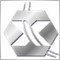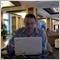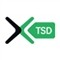Lesson 4 - MQL4 Operations & Expressions21838

Hi folks,

I hope you enjoyed my last 3 lessons.

I didn't hear questions from you yet.

I don't think that everything 100% understood in my lessons.

so, Where are the questions?

I hope to hear you soon.

Files:
lesson4.pdf 101 kbModerator
110862

1. It is written the following:

A = B + C, with description that add A to B and assign the result to C.

Is it mistake?

The same with A = B - C.

2. And I do not understand A = A % C, with description: A is remider of division of B on C(ex: 10%2 will produce 0).

I simple do not understand 10 % 2 = 0 and 10 % 3 = 1.

May you explain? :confused:

3. It was mentioned that we can not write as:

A=(B++)*5

we must write: :confused:

A++;

B=A*5

May be the following:

B++;

A=B*5Moderator
110862

And the other 3 questions.

1. May you explain

A %= B;

Because I do not understand A = A % B

as well.

2. And

A >>= B

Is it

A is greater or equal B ?

3. A += B

it is A = A + B

isn't it?218382183814401440Moderator
110862

Ok. Now I understand.

We can not use our simple arithmetical knowledge.

A += B

is the A increasing on B.

A -= B

is A decreasing on B.

A = B%C

is the remainder from dividing B by C.

And I think A /= B

is

A = A / B.

And A = A + B

is valid because it is not arithmetic.

It is logic.1440

newdigital:

And A = A + B

is valid because it is not arithmetic.

It is logic.

It's arithmetic.

int A=12;

int B=6;

A = A + B; // --> A = 12 +6;Moderator
110862

It is arithmetical operations but it is logic.

In arithmetic if

A + B so it should be C already.

It should not be the same A (the same value).

Because if we are increasing A on B assigning the result to A so

A will be increasing on B all the time. Every second A will be increasing on B without any stopping. It means A plus B is equal A (the same A?) so plus B once again (because of A plus B) is equal A (an other A) which should be added to B and so on.

May be during the coding everything will be clear.

But I have a question.

In Relational operators A == Bi;

It means true if A equal B else false.

Is it necessary to have i after B?1440

newdigital:
It is arithmetical operations but it is logic.

In arithmetic if

A + B so it should be C already.

It should not be the same A (the same value).

Because if we are increasing A on B assigning the result to A so

A will be increasing on B all the time. Every second A will be increasing on B without any stopping. It means A plus B is equal A (the same A?) so plus B once again (because of A plus B) is equal A (an other A) which should be added to B and so on.

May be during the coding everything will be clear.

But I have a question.

In Relational operators A == Bi;

It means true if A equal B else false.

Is it necessary to have i after B?

"A" is only the variable where we store value.

A = A + B; means that we want to modify the value stored in A ... with a new value (A+B).

Of course after executing that struction ... A will have new value.

if(A == B)

{

....

}

i After B is not needed and would be an error.